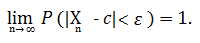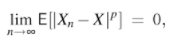# Convergence of Random Variables: Simple Definition

## Convergence of Random Variables

Convergence of random variables (sometimes called stochastic convergence) is where a set of numbers settle on a particular number. It works the same way as convergence in everyday life; For example, cars on a 5-line highway might converge to one specific lane if there’s an accident closing down four of the other lanes. In the same way, a sequence of numbers (which could represent cars or anything else) can converge (mathematically, this time) on a single, specific number. Certain processes, distributions and events can result in convergence— which basically mean the values will get closer and closer together.

When Random variables converge on a single number, they may not settle exactly that number, but they come very, very close. In notation, x (xn → x) tells us that a sequence of random variables (xn) converges to the value x. This is only true if the https://www.statisticshowto.com/absolute-value-function/#absolute of the differences approaches zero as n becomes infinitely larger. In notation, that’s:

|xn − x| → 0 as n → ∞.

What happens to these variables as they converge can’t be crunched into a single definition. Instead, several different ways of describing the behavior are used.

## Convergence of Random Variables: Types

Convergence of Random Variables can be broken down into many types. The ones you’ll most often come across:

Each of these definitions is quite different from the others. However, for an infinite series of independent random variables: convergence in probability, convergence in distribution, and almost sure convergence are equivalent (Fristedt & Gray, 2013, p.272).

## 1. Convergence in probability

If you toss a coin n times, you would expect heads around 50% of the time. However, let’s say you toss the coin 10 times. You might get 7 tails and 3 heads (70%), 2 tails and 8 heads (20%), or a wide variety of other possible combinations. Eventually though, if you toss the coin enough times (say, 1,000), you’ll probably end up with about 50% tails. In other words, the percentage of heads will converge to the expected probability.

More formally, convergence in probability can be stated as the following formula:Where:

• P = probability,
• Xn = number of observed successes (e.g. tails) in n trials (e.g. tosses of the coin),
• Lim (n→∞) = the limit at infinity — a number where the distribution converges to after an infinite number of trials (e.g. tosses of the coin),
• c = a constant where the sequence of random variables converge in probability to,
• ε = a positive number representing the distance between the expected value and the observed value.

The concept of a limit is important here; in the limiting process, elements of a sequence become closer to each other as n increases. In simple terms, you can say that they converge to a single number.

## 2. Convergence in distribution

Convergence in distribution (sometimes called convergence in law) is based on the distribution of random variables, rather than the individual variables themselves. It is the convergence of a sequence of cumulative distribution functions (CDF). As it’s the CDFs, and not the individual variables that converge, the variables can have different probability spaces.

In more formal terms, a sequence of random variables converges in distribution if the CDFs for that sequence converge into a single CDF. Let’s say you had a series of random variables, Xn. Each of these variables X1, X2,…Xn has a CDF FXn(x), which gives us a series of CDFs {FXn(x)}. Convergence in distribution implies that the CDFs converge to a single CDF, Fx(x) (Kapadia et. al, 2017).

Several methods are available for proving convergence in distribution. For example, Slutsky’s Theorem and the Delta Method can both help to establish convergence. Convergence of moment generating functions can prove convergence in distribution, but the converse isn’t true: lack of converging MGFs does not indicate lack of convergence in distribution. Scheffe’s Theorem is another alternative, which is stated as follows (Knight, 1999, p.126):

Let’s say that a sequence of random variables Xn has probability mass function (PMF) fn and each random variable X has a PMF f. If it’s true that fn(x) → f(x) (for all x), then this implies convergence in distribution. Similarly, suppose that Xn has cumulative distribution function (CDF) fn (n ≥ 1) and X has CDF f. If it’s true that fn(x) → f(x) (for all but a countable number of X), that also implies convergence in distribution.

## 3. Almost sure (a.s.) convergence

Almost sure convergence (also called convergence in probability one) answers the question: given a random variable X, do the outcomes of the sequence Xn converge to the outcomes of X with a probability of 1? (Mittelhammer, 2013).

As an example of this type of convergence of random variables, let’s say an entomologist is studying feeding habits for wild house mice and records the amount of food consumed per day. The amount of food consumed will vary wildly, but we can be almost sure (quite certain) that amount will eventually become zero when the animal dies. It will almost certainly stay zero after that point. We’re “almost certain” because the animal could be revived, or appear dead for a while, or a scientist could discover the secret for eternal mouse life. In life — as in probability and statistics — nothing is certain.

Almost sure convergence is defined in terms of a scalar sequence or matrix sequence:

Scalar: Xn has almost sure convergence to X iff: P|Xn → X| = P(limn→∞Xn = X) = 1

Matrix: Xn has almost sure convergence to X iff: P|yn[i,j] → y[i,j]| = P(limn→∞yn[i,j] = y[i,j]) = 1, for all i and j.

## Almost Sure in Convergence vs. Convergence in Probability

The difference between almost sure convergence (called strong consistency for b) and convergence in probability (called weak consistency for b) is subtle. It’s what Cameron and Trivedi (2005 p. 947) call “…conceptually more difficult” to grasp. The main difference is that convergence in probability allows for more erratic behavior of random variables. You can think of it as a stronger type of convergence, almost like a stronger magnet, pulling the random variables in together. If a sequence shows almost sure convergence (which is strong), that implies convergence in probability (which is weaker). The converse is not true — convergence in probability does not imply almost sure convergence, as the latter requires a stronger sense of convergence.

## 4. Convergence in mean.

A series of random variables Xn converges in mean of order p to X if:Where 1 ≤ p ≤ ∞.
When p = 1, it is called convergence in mean (or convergence in the first mean). When p = 2, it’s called mean-square convergence.

Convergence in mean is stronger than convergence in probability (this can be proved by using Markov’s Inequality). Although convergence in mean implies convergence in probability, the reverse is not true.

## References

Cameron and Trivedi (2005). Microeconometrics: Methods and Applications. Cambridge University Press.
Gugushvili, S. (2017). Convergence of Random Variables. Retrieved November 29, 2017 from: http://pub.math.leidenuniv.nl/~gugushvilis/STAN5.pdf
Jacod, J. & Protter, P. (2004). Convergence of Random Variables. In Probability Essentials. Springer.
Kapadia, A. et al (2017). Mathematical Statistics With Applications. CRC Press.
Knight, K. (1999). Mathematical Statistics. CRC Press.
Fristedt, B. & Gray, L. (2013). A Modern Approach to Probability Theory. Springer Science & Business Media.
Mittelhammer, R. Mathematical Statistics for Economics and Business. Springer Science & Business Media.

CITE THIS AS:
Stephanie Glen. "Convergence of Random Variables: Simple Definition" From StatisticsHowTo.com: Elementary Statistics for the rest of us! https://www.statisticshowto.com/convergence-of-random-variables/